# Singular exponents

(diff) ← Older revision | Latest revision (diff) | Newer revision → (diff)

of a linear system of ordinary differential equations

The quantities defined by:(the upper singular exponent) and(the lower singular exponent), where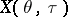is the Cauchy operator (i.e. the fundamental solution or principal solution) of the system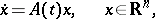(1)

where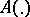is a mapping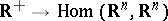that is summable on every interval.

The singular exponents can be equal to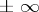; if for a certain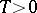,(1prm)

then the singular exponents are numbers.

For a system (1) with constant coefficients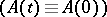, the singular exponents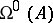and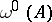are equal to, respectively, the maximum and minimum of the real parts of the eigenvalues of the operator. For a system (1) with periodic coefficients (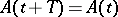for all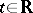for a certain), the singular exponentsandare equal to, respectively, the maximum and minimum of the logarithms of the absolute values of the multipliers, divided by the period. The singular exponents are sometimes also called general exponents (see ).

The following definitions are equivalent to those mentioned above if (1prm) holds for a certain: The singular exponentis equal to the greatest lower bound of the set of those numbersfor each of which there is a number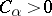such that for any solution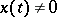of the system (1) the inequality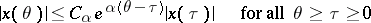is fulfilled; the singular exponentis equal to the least upper bound of the set of those numbersfor each of which a number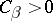exists such that for every solutionof the system (1) the inequality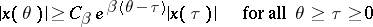is fulfilled.

For the singular exponents and for the Lyapunov characteristic exponents (cf. Lyapunov characteristic exponent), for eachthe inequalities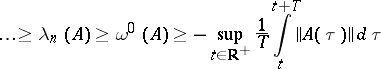hold. For linear systems with constant or periodic coefficients,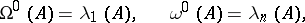but there exist systems for which the corresponding inequalities are strict (see Uniform stability).

The singular exponent(respectively,), as a function on the space of all systems of the form (1) with bounded continuous coefficients (the mappingis continuous and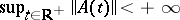) provided with the metricis semi-continuous from above (respectively, from below) but is not continuous everywhere.

If the mappingis uniformly continuous and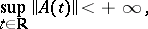then the shift dynamical systemhas invariant normalized measures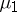andconcentrated on the closure of the trajectory of the pointsuch that, for almost all(in the sense of the measure), the upper singular exponent of the system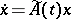(2)

is equal to its largest (leading) Lyapunov characteristic exponent,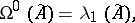and for almost all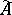(in the sense of the measure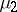), the lower singular exponent of the system (2) is equal to its smallest Lyapunov characteristic exponent,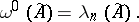For almost-periodic mappings(see Linear system of differential equations with almost-periodic coefficients) the measuresandare identical and coincide with the unique normalized invariant measure concentrated on the restriction of the shift dynamical system to the closure of the trajectory of the point, which in this case exists.

Let a dynamical system on a smooth, closed-dimensional manifold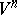be defined by a smooth vector field. Then there exist normalized invariant measuresandfor this system such that for almost every point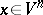(in the sense of the measure) the upper singular exponent and the leading Lyapunov characteristic exponent of the system of variational equations (equations in variations, linearized equations) along the trajectory of the pointcoincide, and for almost every point(in the sense of the measure) the lower singular exponent and the smallest Lyapunov characteristic exponent of the system of variational equations along the trajectory of the pointcoincide. The definitions of singular exponents, Lyapunov characteristic exponents, etc., retain their meaning for systems of variational equations of smooth dynamical systems defined on arbitrary smooth manifolds. The system of variational equations of such a dynamical system along the trajectory of a pointcan be written in the form (1) by using, for example, that basis in the tangent space toat every point of the trajectory ofwhich is obtained by a parallel transfer along this trajectory (in the sense of the Riemannian connection induced by the smooth Riemannian metric) of some basis of the tangent space ofat.

How to Cite This Entry:
Singular exponents. Encyclopedia of Mathematics. URL: http://encyclopediaofmath.org/index.php?title=Singular_exponents&oldid=11292
This article was adapted from an original article by V.M. Millionshchikov (originator), which appeared in Encyclopedia of Mathematics - ISBN 1402006098. See original article